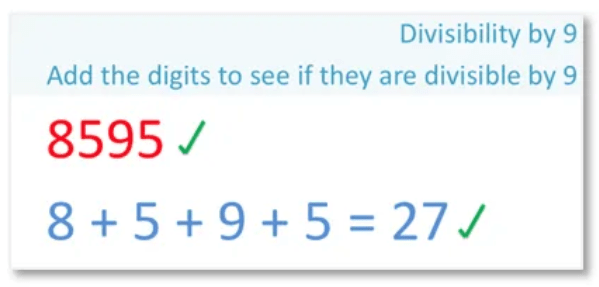# How to Tell if a Number if Divisible by 3

How to Tell if a Number if Divisible by 3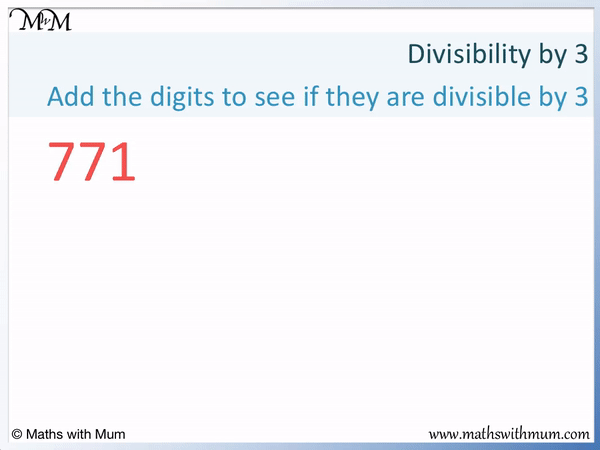• A number is divisible by 3 if the sum of the digits is also divisible by 3.
• If a number is divisible by 3 it means that the number is in the 3 times table.
• Firstly, add the individual digits of a number.
• 7 + 7 + 1 = 15.
• Then check to see if this answer is in the 3 times table.
• 15 is in the 3 times table and so 771 is also in the 3 times table.
• 771 is divisible by 3.
• This divisibility by 3 rule works for every number.
• The rule can be applied to the result of sum of the digits to check that too.
• In this example, we know that 15 is divisible by 3 because 1 + 5 = 6 and 6 is divisible by 3.
Add the digits of the number.

If this answer is divisible by 3, the original number is divisible by 3.• The rule for divisibility by 3 works for all numbers no matter how large.
• Add the digits of the number and check if this result is also divisible by 3.
• We add the individual digits of the number 7, 749, 984.
• 7 + 7 + 4 + 9 + 9 + 8 + 4 = 48.
• We might not be sure if 48 is divisible by 3.
• The rule can be applied again to 48 to see if 48 is divisible by 3.
• 4 + 8 = 12, which is in the 3 times table.
• 12 is in the three times table, therefore so is 48 and so is 7, 749, 984.
• 7, 749, 984 is divisible by 3, which means that 7, 749, 984 is in the 3 times table.Supporting Lessons# How to Tell if a Number is Divisible by 3

## How to Tell if a Number is Divisible by 3

To test if a number is divisible by 3, follow these steps:

1. Add the individual digits of the number to make a total.
2. If this total is divisible by 3, the original number is divisible by 3.
3. If you are not sure if the total is divisible by 3, apply the first two steps to that number.

If a number is divisible by 3, it means that the number is in the 3 times table. A number that is divisible by 3 is a multiple of 3. The number can be divided exactly by 3 to leave no remainder.

In this example, we will use the divisibility by 3 rule to test 5502. Is 5502 in the 3 times table?

A number is divisible by 3 if the sum of its digits is also divisible by 3. For example, 5502 is divisible by 3 because 5 + 5 + 0 + 2 = 12. 12 is divisible by 3 and so, 5502 is divisible by 3.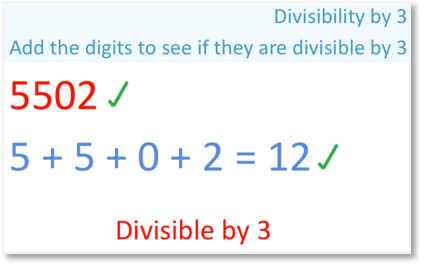The first step is to add the digits of the number.

5 + 5 + 0 + 2 = 12.

The next step is to check if this new, smaller number is divisible by 3.

12 is 4 × 3. 12 is in the three times table and so, 5502 is also in the three times table.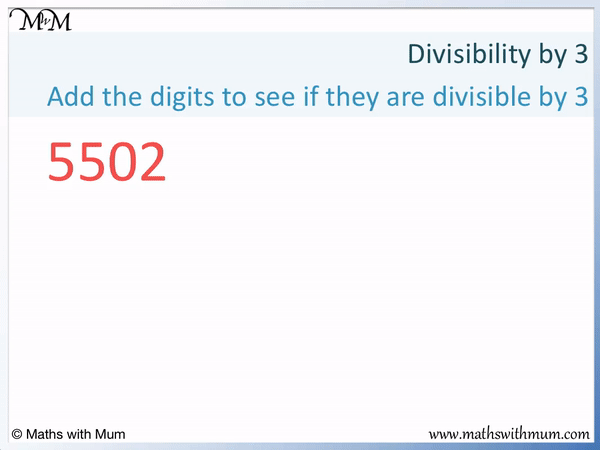We can also check that each number in the working out is a multiple of 3 by adding its digits.

12 is a multiple of 3 because 1 + 2 = 3.

Here is another example of using the rule for divisibility by 3 to test if a number is a multiple of 3.

Is the number 409 a multiple of 3?The first step is to add the digits of the number.

4 + 0 + 9 = 13.

The next step is to decide if the sum of the digits is a multiple of 3.

13 is not a multiple of 3. It is not in the 3 times table. We can also see that 1 + 3 = 4 and 4 is not a multiple of 3, therefore 13 is not a multiple of 3.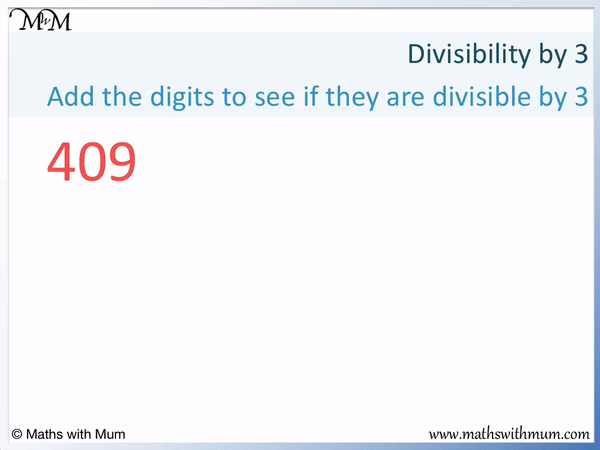13 is an example of a number that is not divisible by 3 and so, 409 is not divisible by 3.

A number is not divisible by 3 if the sum of its digits is not divisible by 3. 409 is not divisible by 3 because 4 + 0 + 9 = 13 and 13 is not divisible by 3.

Prime numbers are not divisible by 3 because they are ony divisible by 1 and themselves. For example, 13 is a prime number and so it not divisible by 3.

The rule for divisibility by 3 works for all numbers no matter how large.

For example, here is the number 529, 943.

The first step is to add the digits of the number.

5 + 2 + 9 + 9 + 4 + 3 = 32The next step is to test if the sum of the digits is divisible by 3.

32 is not divisible by 3. We know this because 30 and 33 are multiples of 3 and 32 is in between these numbers.

We can also use the same test on 32 to show that it is not divisible by 3. We add the digits. 3 + 2 = 5 and 5 is not a multiple of 3.

32 is not a multiple of 3 and therefore 529, 943 is not a multiple of 3 either. 529, 943 is an example of a number that is not divisible by 3.

Here is an example of testing a large number to see if it is in the 3 times table.

Is 7, 749, 984 a multiple of 3?

7 + 7 + 4 + 9 + 9 + 8 + 4 = 48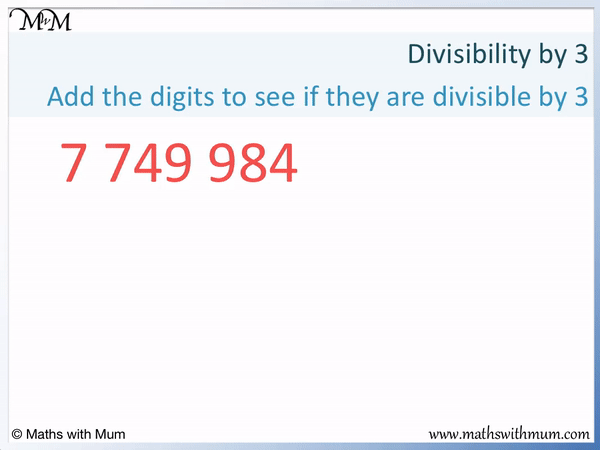48 is 16 × 3 and so, it is a multiple of 3.

If we were not sure if the number was a multiple of 3, add the digits and see if the total is a multiple of 3.

4 + 8 = 12, which is 4 × 3. 12 is a multiple of 3 and so, 48 is a multiple of 3 and 7, 749, 984 is also a multiple of 3

## Why Does the Divisibility Rule for 3 Work?

The divisibility rule for 3 works because the number represented by each digit can be written as a multiple of 9 plus that digit. 9 is divisible by 3 so if the sum of the digits is divisible by 3, the number itself is too.

Here is the proof that 3174 is divisible by 3.

The digit 3 stands for 3000, which is 1000 × 3.

This is the same as 999 × 3 plus one more 3.

The digit 1 stands for 100, which is 100 × 1.

This is the same as 99 × 1 plus one more 1.

The digit 7 stands for 70, which is 10 × 7.

This is the same as 9 × 7 plus one more 7.

The digit 4 stands for one lot of 4.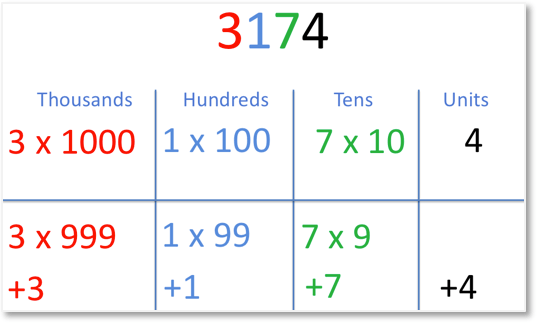The multiples of 9, 99 and 999 are all divisible by 3.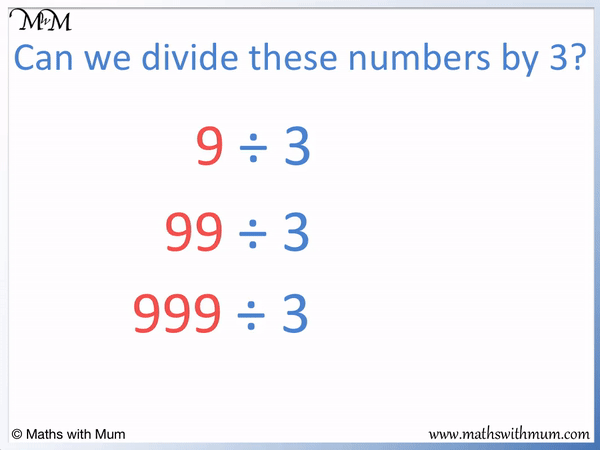3174 is equal to 999 × 3 + 99 × 1 + 9 × 7 plus 3 + 1 + 7 + 4.

The multiples of 9, 99 and 999 are all divisible by 3, so we only need to check the sum of the digits: 3 + 1 + 7 + 4.3 + 1 + 7 + 4 = 15, which is divisible by 3.

Since the multiples of 9, 99 and 999 along with the sum of the digits are all divisible by 3, the entire number is divisible by 3.

All numbers are written in base 10. This means that the digits of each number represent a multiple of 9 plus that digit. The multiples of 9 are divisible by 3, so we simply need to test the sum of the digits to see if the whole number is divisible by 3.

No matter what the number, add the digits to check if it is divisible by 3.

## List of Numbers Divisible by 3

Here is a list of 2-digit numbers less than 100 that are divisible by 3:

3, 6, 9, 12, 15, 18, 21, 24, 27, 30, 33, 36, 39, 42, 45, 48, 51, 54, 57, 60, 63, 66, 69, 72, 75, 78, 81, 84, 87, 90, 93, 96 and 99.

There are 33 2-digit numbers that are divisible by 3. The largest 2 digit number divisible by 3 is 99, which is 33 × 3.

This list can help us to identify some common multiples of 3, which helps us to identify if a larger number is divisible by 3.

Prime numbers are not be divisible by 3. This is because prime numbers can only be divided by 1 and themselves.

Even numbers can be divisible by 3. For example, the even number of 12 is divisible by 3.

All numbers that are divisible by 9 are also divisible by 3. This is because 3 divides exactly into 9.Now try our lesson on Rule for Divisibility by 9 where we learn how to test if a number is divisible by 9.